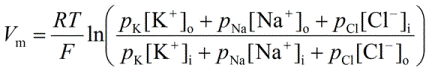PhysiologyWeb  Home  |  FAQ  |  About  |  Contact  |   - Sign InPhysiology Quiz -
The Membrane Potential - Part 2
Cells of multicellular organisms such as animals and plants, as well as those of unicellular organisms such as yeast exhibit a potential difference across the cell plasma membrane. That is to say that there is an electrical potential difference between the inside of the cell and the surrounding bathing medium of the cell. This potential difference is referred to as the membrane potential (Vm). The numerical value of the membrane potential is generally negative, meaning that the inside of the cell is negative with respect to the outside solution, which is taken as the reference or zero value.

Test your knowledge of the membrane potential by taking this series of quizzes. For more information about the membrane potential, see the Resting Membrane Potential lecture notes.
(1)   Which of the following equations is the Nernst equation?
 (A)(B)(2)   Which of the following equations is the Goldman-Hodgkin-Katz (GHK) equation?
 (A)(B)(3)   The following equation should be used to calculate the equilibrium potential for any given ion.
 (A)   The Nernst equation(B)   The Goldman-Hodgkin-Katz (GHK) equation(4)   For a typical cell, the following equation should be used to calculate the membrane potential.
 (A)   The Nernst equation(B)   The Goldman-Hodgkin-Katz (GHK) equation(5)   Based on physiological concentrations of K+, Na+, and Cl (see here), determine the resting membrane potential in a typical neuron. Assume that pK = 1, pNa = 0.05, and pCl = 0.5.
 (A)   −68 mV (B)   +68 mV (C)   0 mV (D)   −70 mV (E)   −90 mVPosted: Tuesday, August 12, 2014
Last updated: Monday, August 11, 2014